# Atmega8 PWM control(frequency,polarity,duty cycle) tutorial with example program

If You come there from internet search i assume You already know what is PWM and where it can be used, so in this post I’ll only explain how to use 8 bit timer in Atmega8 to generate PWM signal and control frequency, polarity and duty cycle.

PWM can be generated from 16-bit Timer/Counter1 or 8-bit Timer/Counter2 but this time I’ll only explain 8-bit Timer/Counter2 Fast PWM Mode.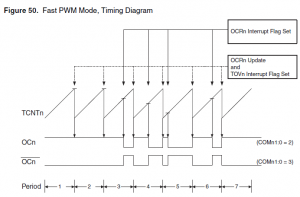In this picture from Atmega8 documentation Fast PWM mode is explained.  Counter(8bit) counts from 0x00 to 0xFF and restarts from 0x00. In not inverting mode OC2 is cleared when counter match value in OCR2 register and set at 0x00. In inverting mode OC2 is set when counter match value in OCR2 register and cleared at 0x00.

From this all turns out that PWM duty cycle depends on OCR2 register. In not inverting mode duty cycle = OCR2/256*100% and it’s  50% if OCR2 is 0x80(middle between 0x00 – 0xFF).

In every PWM period counter must count 256 steps, so frequency of signal is 256 times lower than counter clock from prescaler. PWM frequency = Atmega clock frequency/timer prescaler(1,8,32,64,128,256)/256. With 4 MHz crystal maximum(without prescaler) PWM frequency is 15 625Hz.

Register setup

For example 15 625 Hz, 50 % duty cycle, non-inverting mode PWM signal generation.

1. OCR2=0x80(128); As mentioned before duty cycle = OCR2/256*100%

2. TCCR2=0x69;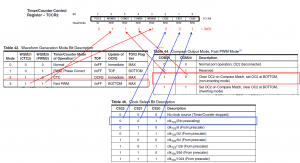3. DDRB=0x08; PWM signal is outputted by toggling  level of PORTB pin 3.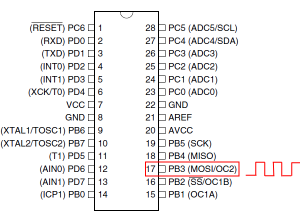DDRB sets it as output.

All together:

```#include <iom8.h> int main( void ) { DDRB=0x08;```

` `

```OCR2=0x80; TCCR2=0x69; while (1) {}; }```

Result: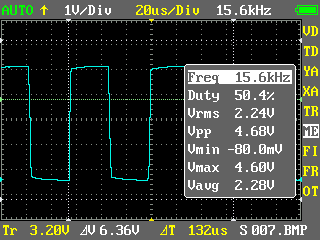~10% duty cycle, 61 Hz.

```#include <iom8.h> int main( void ) { DDRB=0x08; OCR2=0x1A; // 256/10=25,6 26 in hex = 1A; TCCR2=0x6E; // 256 prescaler ```

` `

```while (1) {}; }```

Result: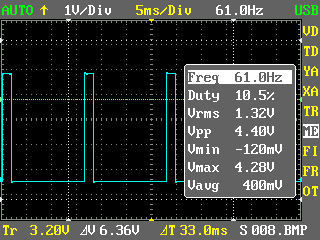And there is demo program and *.hex file for PWM demo seen in video.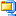Atmega8 PWM demo program (1.8 KiB, 9,038 hits)

# Arduino 4 digits 7 segments LED countdown timer with buzzer

After sorting out  how works 16 bit hardware timer it is time for 4 digits countdown timer. Having 16 bit timer and  7 segments LED code from earlier only were remaining to write timer’s modes (run/setup) and button’s control code.  After putting  all code to one place there is countdown timer with properties below:

• Maximum 99 minutes 59 seconds countdown interval
• 1 second resolution
• Sound indication with buzzer for finished countdown
• LED indicates running timer, or relay instead  for powering external devices for some period of time
• 2 buttons to set timer and start/pause/reset.

This time without additional code quotation, please find some code explanation within code, so code bellow.

# 4 digits, 7 segments LED display multiplexing with Arduino

Last time I showed You how to control 1 digit 7 segment LED display with Arduino. This time it’s not 1, but 4 digits. To connect 1 digit to Arduino we had to use 8 ports, so to connect 4 digits we need to have 4×8=32? Not necessary. Where is a way to use much less ports, it’s called multiplexing. Using multiplexing at one time only one digit is active(e.g. for 2ms). All digits is turned on is serial, but because human’s eye is inert we have illusion, that all digits are lighting at same time.

As You can see form schematic bellow with multiplexing implemented we required only 4 additional ports compared to 1 digit circuit, total – 12.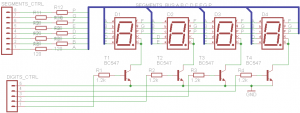Because at the same time only one digit will be on All 4 digits segments inputs are connected together. By connecting digits common cathodes to ground we are controlling which digit shall be turned on. Atmega 1280 µCPU port can drain(receive) maximum 40 mA current. If all one digits segments are on, we are having 20×8= 160 mA that is to much, so we can’t to connect common cathodes directly to Arduino ports.  Therefore I have used BC547 NPN transistors as switches. Transistor is opened, when positive voltage is applied at the base.

Let’s see the code.

# Controlling 7 segment LED display from Arduino. Full code included.

So this is first real electronics mini project/tutorial in this blog.

Everybody at the beginning usually shows how to turn on one LED,  I do it a little more complex by controlling 7 segment LED display.

For this demonstration I use one Kingbright SC56-11EWA module with common cathode, it means that all 8 (7 segments +  1 dot) LED’s cathode are connected together: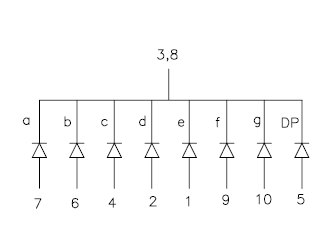The first step is to connect 7 segment display LED’s anodes to Arduino ports. You need 7 free ports, or 8 if You want use “dot”. Display datasheet comes very handy, when You need to found out which pin is which.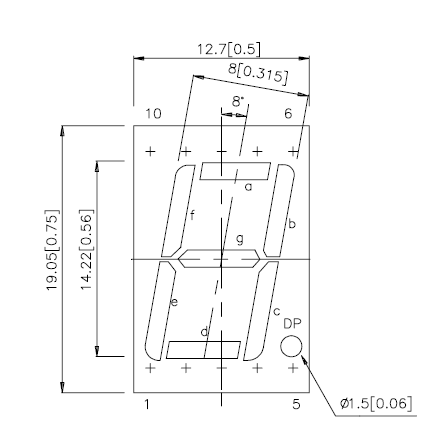Do not forget to connect LED’s pins with resistors in serial. Typical red LED forward voltage is 2.0V @20mA current, but Arduino ports outputs 5V, so You must use resistor, in this situation (5-2)/0.02= 150 Ohm or higher should be ok.

Common cathode (3 and/or 8 pin) should be connected to GND and if You have display with common anode You should connect it to +5V.

So if everything is connected we should start to analyze program code.

To make life easier I have done same definitions, so after that I don’t need to remember which Arduino ports I selected to connect display’s segments:

```#define G 22 #define F 23 #define A 24 #define B 25 #define E 26 #define D 27 #define C 28 #define DP 29```

Arduino ports can be outputs (e.g. for controlling LED) or inputs (e.g. to detect pressed button, sense voltage). We need outputs to turn on LED’s so:

```pinMode(A, OUTPUT); pinMode(B, OUTPUT); pinMode(C, OUTPUT); pinMode(D, OUTPUT); pinMode(E, OUTPUT); pinMode(F, OUTPUT); pinMode(G, OUTPUT); pinMode(DP, OUTPUT);```

To display digit on display we need to turn on some segments accordingly to digit.

For example for “7” we need to turn on A B C segments and other segments should be turned off.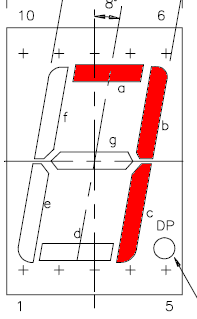So where is function for what as and for all other digits:
`void digit7 () {`

` `

```digitalWrite(A,HIGH); digitalWrite(B, HIGH); digitalWrite(C, HIGH); digitalWrite(D, LOW); digitalWrite(E, LOW); digitalWrite(F, LOW); digitalWrite(G, LOW); }; ```

I also have made a function to display digit form variable, it is very useful in main loop.

```void showdigit (int digit) { switch (digit) { ``````case 0: digit0 (); break; ... case 9: digit9 (); break; default: break; };```

And  the main program. Using for loop as counter from 0 to 9. You see how `showdigit` function is good here, it shows variable “i” content on display.

```for (int i=0;i<10;i++) { //counting from 0 to 9 showdigit(i); delay (1000); // 1000ms= 1s delay if (i%2) { digitalWrite(DP, HIGH); } else {digitalWrite(DP, LOW); };```

If condition is here to check if variable “i” is even and if so shows dot on the display.

Completed code: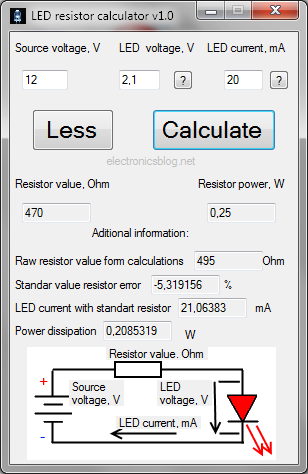This is the very first and I hope not the last post of this blog.  With this event I want to share with you LED calculator. It is also my first project with Visual C++ Expression 2008, this  software development environment is free for students.

Calculator is capable of finding suitable standard E12 (10%) resistor value and power for one LED designs.  There also some tips for user to find LED forward voltage: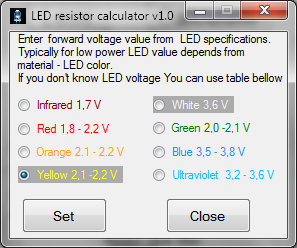Calculator works on Windows XP, Vista, 7, but you have to install Microsoft .NET Framework 3.5LED resistor calculator v1.0 (56.1 KiB, 23,240 hits)